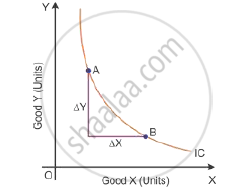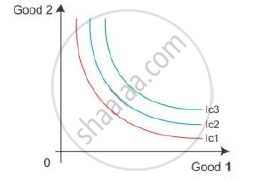# Explain the Three Properties of the Indifference Curves. - Economics

Explain the three properties of the indifference curves.

State three properties of indifference curves

#### Solution

Properties of indifference curves (ICs)

1. Indifference curves slope downwards or negative slope:-

The indifference curves slope downwards, left to right, because an increase in the amount of Good X along the indifference curve is associated with a decrease in the amount of Good Y, as the preferences are monotonic.

2. A slope of indifference curves represents a marginal rate of substitution:-

The marginal rate of substitution (MRS) is the rate at which a consumer is willing to substitute one commodity for another commodity.The slope of indifference curve between A and B =(DeltaY)/(DeltaX)=MRS

MRS is the rate at which the output of Good Y is sacrificed for every additional unit of Good X.

3. In an indifference map, higher IC represents a higher level of satisfaction:-

An indifference map refers to a set of indifference curves. An indifference curve which is to the right and above another shows a higher level of satisfaction to the consumer. Here, IC3 shows the higher level of satisfaction than IC2. Thus, the indifference curve relates to a higher level of income of the consumerConcept: Indifference Curve
Is there an error in this question or solution?
2015-2016 (March) All India Set 1
Share